Math Olympiad Test: Circles- 1

# Math Olympiad Test: Circles- 1

Test Description

## 10 Questions MCQ Test Mathematics Olympiad for Class 9 | Math Olympiad Test: Circles- 1

Math Olympiad Test: Circles- 1 for Class 9 2022 is part of Mathematics Olympiad for Class 9 preparation. The Math Olympiad Test: Circles- 1 questions and answers have been prepared according to the Class 9 exam syllabus.The Math Olympiad Test: Circles- 1 MCQs are made for Class 9 2022 Exam. Find important definitions, questions, notes, meanings, examples, exercises, MCQs and online tests for Math Olympiad Test: Circles- 1 below.
Solutions of Math Olympiad Test: Circles- 1 questions in English are available as part of our Mathematics Olympiad for Class 9 for Class 9 & Math Olympiad Test: Circles- 1 solutions in Hindi for Mathematics Olympiad for Class 9 course. Download more important topics, notes, lectures and mock test series for Class 9 Exam by signing up for free. Attempt Math Olympiad Test: Circles- 1 | 10 questions in 10 minutes | Mock test for Class 9 preparation | Free important questions MCQ to study Mathematics Olympiad for Class 9 for Class 9 Exam | Download free PDF with solutions
 1 Crore+ students have signed up on EduRev. Have you?
Math Olympiad Test: Circles- 1 - Question 1

### The largest chord of a circle is called its:

Detailed Solution for Math Olympiad Test: Circles- 1 - Question 1

The largest chord of a circle is called diameter of the circle.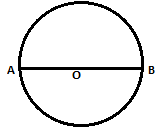Any chord which crosses the centre of the circle is the largest chord of the circle, which is the diameter of the circle.

Math Olympiad Test: Circles- 1 - Question 2

### AB and CD are two parallel chords of a circle such that AB = 8 cm, and CD = 6cm. If the chords are on the opposite sides of the center and the distance between them is 7cm, then the diameter of the circle is:

Detailed Solution for Math Olympiad Test: Circles- 1 - Question 2

Let the radius of the circle be r, and the length OP be x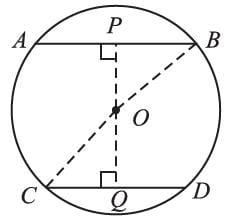∴ In ∆OPB,
OP2 + PB2 = OB2 = r2
⇒ x2 + (4)2 = r  [∵ PB = AB/2]
⇒ x2 = r2 – 16 ….(i)
In ∆OCQ,
OQ2 + CQ2 = r2
⇒  (7 – x)2 + (3)2 = r2
[∵ OQ = PQ - OP = 7 - x]
⇒ (7 – x)2 = r2 – 9 ….(ii)
Subtracting (ii) from (i), we get
x2 – (7 – x)2 = – 7
⇒ (x – 7 + x) (x + 7 – x) = – 7
⇒    (2x – 7) (7) = – 7
⇒  (2x – 7) = – 1
⇒ x = 3
∴ r2 = x2 + 16 [using (i)]
= (3)2 + 16 = 25
⇒ r = 5cm
∴ d = 10 cm.

Math Olympiad Test: Circles- 1 - Question 3

### Two circles of radii 13cm and 15cm intersect and the length of the common chord is 24 cm, then the distance between their centers is:

Detailed Solution for Math Olympiad Test: Circles- 1 - Question 3

In ∆OPA,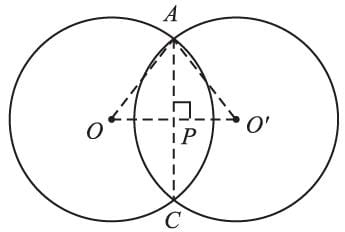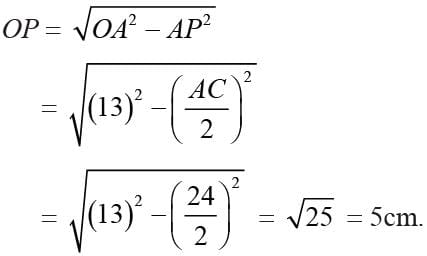Similarly,
In ∆O′PA,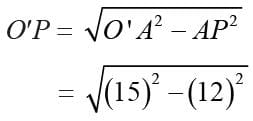= 9cm.
∴ OO′ = OP + O′P = 5 + 9 = 14 cm.

Math Olympiad Test: Circles- 1 - Question 4

Bisector AD of ∠BAC of ∆ABC passes through the center of the circumcircle of ∆ABC, then,

Detailed Solution for Math Olympiad Test: Circles- 1 - Question 4

∵ AD is the bisector of ∠BAC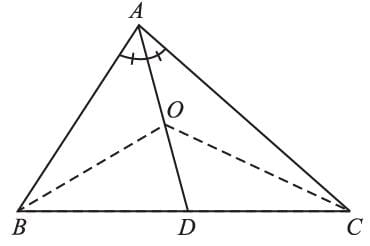∴ AD is the ⊥ bisector of BC.
∵ O is the circumcentre of ∆ABC.
∴ AB = AC [∵ ∠ABD = ∠ACB]
(By using ∆ABD ≅ ∆ACD)

Math Olympiad Test: Circles- 1 - Question 5

Find the measure of ∠ABC.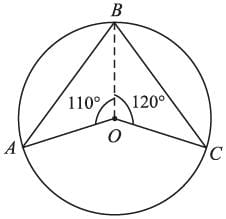Detailed Solution for Math Olympiad Test: Circles- 1 - Question 5

Reflex ∠AOC = 360° – (110° + 120°)
= 130°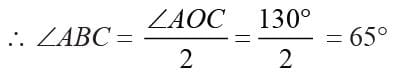Math Olympiad Test: Circles- 1 - Question 6

The measure of ∠BOC is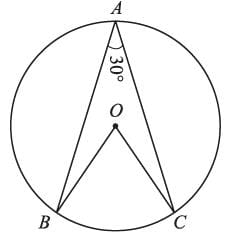Detailed Solution for Math Olympiad Test: Circles- 1 - Question 6

∠BOC = 2 × ∠BAC [Angle subtended at center is double the angle subtended the circle]
= 2 × 30° = 60°

Math Olympiad Test: Circles- 1 - Question 7

P is the center of the circle, and ∠XPZ = 120°, ∠XZY = 35°, then the measure of ∠YXZ is: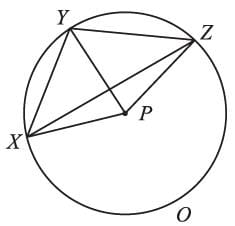Detailed Solution for Math Olympiad Test: Circles- 1 - Question 7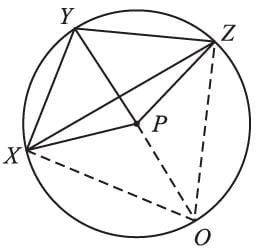∠XPY = 2∠XOP
∵ ∠XOP = ∠XZY
(angles in the same segment)
∴ ∠XPY = 2∠XZY …(i)
Similarly,
∠YPZ = 2∠YXZ …(ii)
Using (i) and (ii)
∠XPZ = 2 (∠XYZ + ∠YXZ)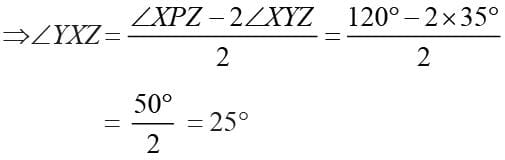Math Olympiad Test: Circles- 1 - Question 8

PQRS is a cyclic quadrilateral such that PR is the diameter of the circle. If ∠QPR = 64° and ∠SPR = 31°, then, ∠R =?

Detailed Solution for Math Olympiad Test: Circles- 1 - Question 8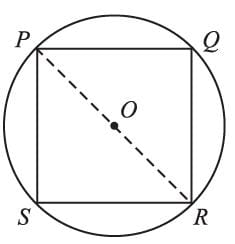∠P = ∠QPR + ∠SPR
= 64 ° + 31°
= 95°
∵ ∠Q and ∠S are angles of the semicircle
∴ ∠Q = ∠S = 90°
∵ PQRS is a cyclic quadrilateral
∴ ∠P + ∠R = 180°
⇒ ∠R = 180° - 95° = 85°

Math Olympiad Test: Circles- 1 - Question 9

If A, B, C are three points on a circle with center O such that ∠AOB = 90° and ∠BOC = 120°, then ∠ABC =?

Detailed Solution for Math Olympiad Test: Circles- 1 - Question 9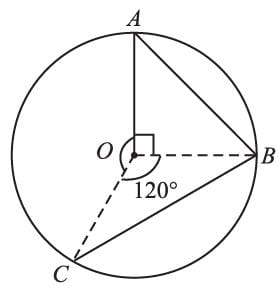Reflex ∠AOC = 360° – (90° + 120°) = 150°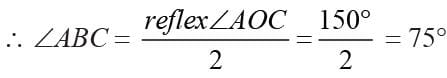Math Olympiad Test: Circles- 1 - Question 10

O is the center of the circle, with AC = 30 cm and DA = 10√5 cm, then the measure of DC is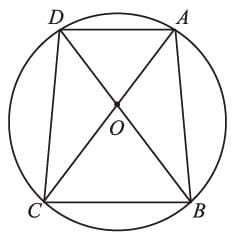Detailed Solution for Math Olympiad Test: Circles- 1 - Question 10

In ∆ACD,
∠ADC = 90° [∵∠ACD is angle in semicircle]
∴  AC2 = DA2 + DC2
⇒ (30)2 = (10√5 )2 + DC2
⇒ DC2 = 900 – 500
⇒ DC = √400 = 20 cm.

## Mathematics Olympiad for Class 9

1 videos|43 tests
 Use Code STAYHOME200 and get INR 200 additional OFF Use Coupon Code
Information about Math Olympiad Test: Circles- 1 Page
In this test you can find the Exam questions for Math Olympiad Test: Circles- 1 solved & explained in the simplest way possible. Besides giving Questions and answers for Math Olympiad Test: Circles- 1, EduRev gives you an ample number of Online tests for practice

## Mathematics Olympiad for Class 9

1 videos|43 tests